# Which DAX formula should you use?

You have a Power BI model that contains the following two tables: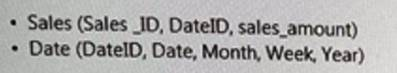The tables have a relationship. Date is marked as a date table in the Power B1 model.

You need to create a measure to calculate the sales for the last 12 months.

Which DAX formula should you use?

A)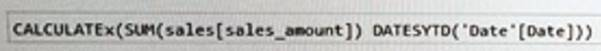B)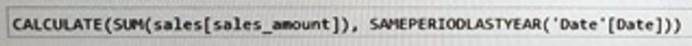C)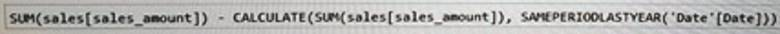D)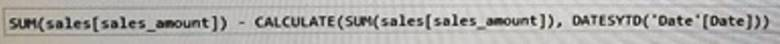A . Option A
B . Option B
C . Option C
D . Option D